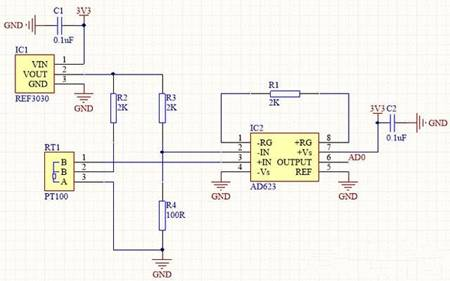﻿ Design of PT100 Temperature Sensing Circuit_

China sensor manufacturers

# Design of PT100 Temperature Sensing Circuit

PT100 is a positive temperature coefficient thermistor. As the temperature increases, the resistance of the resistor becomes larger, which is the positive temperature coefficient of the thermistor. Conversely, if the resistance of the resistor becomes smaller as the temperature rises, it is a thermistor with a negative temperature coefficient.
The PT100 is widely used not only because it can measure a wide temperature range (a few tens of degrees below zero to a few hundred degrees above zero), but also because its linearity is very good. "Linearity" means that each time the temperature changes by one degree, the magnitude of the resistance increase is substantially the same. This greatly simplifies our programming.However, PT100 also has its shortcomings, that is, for every degree of temperature rise, the resistance change is too small, only 0.39 ohms. In this way, it is necessary to provide high precision and low noise conversion on hardware. There are many PT100 design circuits circulating on the Internet, many of which can not be used as product design. Below to provide you with a high-precision circuit, but the cost is somewhat high, but the quality is good.For temperature measurement circuits, there are actually many places worth studying, and small circuits have great wisdom.
For example, can you see at a glance that this circuit can't measure the temperature under zero?
Can you calculate the temperature range from which this circuit can measure?
Can you modify this circuit so that it can measure the temperature range you need?
What happens if you swap the two lines of inverting (-IN) and in-phase (+IN)?

Look, you think the circuit is simple, then the above questions can be answered?

Pt100 circuit explanation:
The simpler the circuit, the better the stability. All four resistors in this circuit require 0.1% accuracy. The circuit uses only one bridge and one differential amplifier. R2 R3 R4 and PT100 form a bridge circuit, and REF3030 provides a standard 3.00V voltage for the bridge circuit. The AD623 uses a 2K amplified feedback resistor to accurately amplify the bridge's differential voltage by a factor of 51.
(Why is it 51 times, see the AD623 datasheet for details)

PT100 circuit wiring method:
Attentive little partners will study the wiring method of PT100. PT100 generally has two and three-wire sensors. Because the line itself must have resistance, and as mentioned above, the PT100 only changes 0.39 ohms for every change. Then if the line of PT100 is very long, the resistance will be larger, the line will be different, and the resistance will be different, which will definitely affect the measured result. So, you can understand now, the two-wire PT100 is only suitable for short-distance applications. Let's see how the three-wire system eliminates the effect of resistance on temperature. Forget it, let's talk about it in the next article. It is necessary to draw a few pictures to make it clear.

PT100 temperature range:
Assuming that it is now 0 degrees, the resistance of PT100 is 100 ohms. In the circuit, the voltage difference of the bridge is 0V, so the last is also 0V, that is, when 0V is measured, it is 0 degrees. Assuming zero now, the resistance of PT100 is less than 100 ohms. The voltage of the same phase will be smaller than the voltage of the inversion, and the obtained voltage will always be 0V, so this circuit will not measure below 0 degrees.

The AD623 has a maximum output of 3.3V and 3300/51 = 64.7mV. That is to say, the voltage difference of the bridge can only be 64.7mV at the maximum, and the large differential pressure, the output of the AD623 is also 3.3V at the maximum. The voltage of the inverting arm is fixed at (3000/2100)*100=142.86mV, so the voltage of the same phase arm can only be 142.86+64.7=207.56mV, and the resistance of PT100 is equal to 207.56/((3000-207.56)/ 2000) = 148.66 ohms.Then look up the table, you can see that the maximum temperature measurement point is almost 127 degrees. Therefore, the temperature range of this circuit is 0~127 degrees.

﻿
﻿